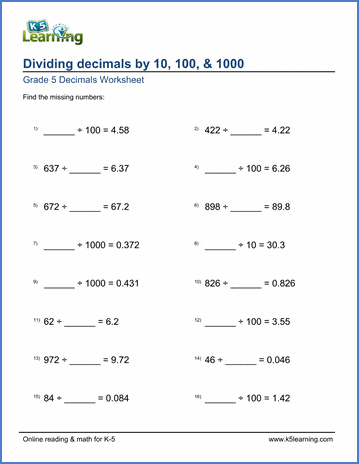# Decimal Division

## Dividing with decimals worksheets for grade 5

Our grade 5 decimal division worksheets start with simple "mental math" questions emphasizing the understanding of decimal place value and finish with more challenging decimal long division exercises.

## Decimal division

Divide decimals by whole numbers 8.8 ÷ 2 =___
Divide decimals by whole numbers (missing number) ___ ÷ 2 = 4.4

Divide 2-digit decimals by whole numbers 0.84 ÷ 7 =___
Divide 2-digit decimals by whole numbers (missing number) 0.84 ÷ __ = 0.12

Decimal divided by decimal (1 digit) 4.2 ÷ 0.7 =
Decimal divided by decimal (2 digits) 0.14 ÷ 0.07 =

## Dividing by 10, 100 or 1000

Dividing whole numbers by 10, 100 or 1000 592 ÷ 100 =
Divide by 10, 100 or 1000 (missing numbers) ___ ÷ 100 = 5.92

Divide 1-digit decimals by 10 or 100 4.6 ÷ 100 =
Divide 1-digit decimals by 10, 100 or 1000 7.7 ÷ 1000 =

## Decimal long division

Divide decimals by whole numbers (1-9) 0.224 ÷ 7 =
Divide decimals by whole numbers (1-99) 9.24 ÷ 70 =

Divide decimals by whole numbers, with rounding 64.8 ÷ 7 =
Divide whole numbers, with rounding 674 ÷ 6 =

Divide whole numbers by decimals 558 ÷ 0.03 =
Long division of decimals by decimals 77.4 ÷ 0.3 =

## Money notation

Divide decimals with money notation \$77.40 ÷ 9 =Sample Grade 5 Decimal Division Worksheet

## More decimals worksheets

Find all of our decimals worksheets, from converting fractions to decimals to long division of multi-digit decimal numbers.

What is K5?

K5 Learning offers free worksheets, flashcards and inexpensive workbooks for kids in kindergarten to grade 5. Become a member to access additional content and skip ads.